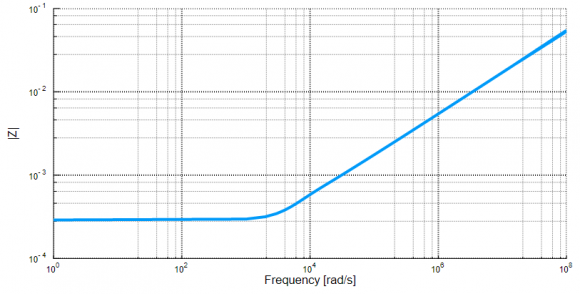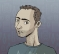## Inverse FFT of a Sampled Frequency Domain FunctionStarted by 4 years ago5 replieslatest reply 4 years ago469 views

Pardon the basic nature of this question.  DSP is not my area of expertise.  I have an equation for a continuous frequency domain function - I'll call it Z(jω) - see embedded plot of the magnitude of this function.  Using the Julia programming language (similar to Python) I first create a discrete array of uniformly spaced frequencies (from ω=0 to ω=1e8 in increments of Δω=1000 ). I then evaluate Z(jω) at each frequency.  The result is a discrete array of complex numbers.  I would like to take the inverse FFT of this data to find the corresponding time domain function.  The IFFT routine is similar to that in Python or Matlab.

• I can apply the IFFT function directly to the array data (see second plot), but should I be conditioning the input array before doing so?
• Also is there typically some kind of scaling that happens as part of the IFFT process?  The output magnitude quite a bit smaller than I expected.

Z(jω)Z(n) after direct application of IFFT to the data sampled from Z(jω) between ω=0 and 1e8[ - ]There's no need to condition the input array.  Doing so would be a convolution with the conditioning window and the Z() function.  There are different approaches to magnitude changes with FFT routines.  One is to put a $1/sqrt(n)$ in front of the FFT and IFFT, the other is to put $1/n$ in front of the IFFT only.  You'll have to look into the details of how Julia does it.

[ - ]Thanks!  If I proceed to process the input array directly, am I correct in my understanding that only the first half of the resulting time array is "useful"?

[ - ]Like drmike said you first of all have to see how Julia implements the routine to make sure you can "compensate" for the magnitude "inconsistencies" (first of all you have to make sure THERE IS an error though 8|).

Now, regarding your time array... The answer is no! This kinda works "the other way around". That means that for a real-valued time domain signal your Fourier transform's magnitude will be an even function (with respect to zero - or Nyquist - frequency, check the implementation for that. Matlab mirrors against Nyquist) and the phase will by an odd function (with respect to the same axis). This means that you could keep half the array to know what's the magnitude and phase content of your signal.

REMINDER: This is true ONLY for real valued functions!

The inverse does not work like that! You need the full Fourier vector (complex numbers) to go back to the time domain. This being said, in case you have only half of it means that you will have to create the rest. That is, mirror your magnitude response and mirror and negate the phase response. Also you should not forget to convert from polar to Cartesian coordinates if needed as most IFFT routines expect real and imaginary numbers instead of magnitude and phase values.

Hope that helps a bit...

[ - ]My data is in Cartesian so it sounds like I need to create a complex conjugate array, reverse the order and prepend to the existing array.  Thanks!

[ - ]Hi jleman.
Assumming your FFT software only works when the number of time samples is an integer power of two, perform the following test:

(1) Make sure your original positive-frequency vector of samples contains M+1 samples where M is an integer power of two. For example, with M = 4 let's say your positive-frequency magnitude and phase samples are:

Mag = [9, 3.3571, 8.0623, 22.6877   27]
Phase = [0, -1.1194, -0.1244, -1.1851, 0]